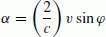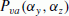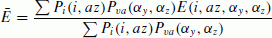# Asymmetric dihedral angle offsets for large-size lunar laser ranging retroreflectors

## Abstract

The distribution of two-dimensional velocity aberration is off-centered by 5 to 6 microradians in lunar laser ranging, due to the stable measurement geometry in the motion of the Earth and the Moon. The optical responses of hollow-type retroreflectors are investigated through numerical simulations, especially focusing on large-size, single-reflector targets that can ultimately minimize the systematic error in future lunar laser ranging. An asymmetric dihedral angle offset, i.e. setting unequal angles between the three back faces, is found to be effective for retroreflectors that are larger than 100 mm in diameter. Our numerical simulation results reveal that the optimized return energy increases approximately 3.5 times more than symmetric dihedral angle cases, and the optimized dihedral angle offsets are 0.65–0.8 arcseconds for one angle, and zeroes for the other two angles.

## 1. Introduction

Laser ranging has been the most precise method to directly measure the Earth-Moon distance since the Apollo-Lunokhod era. Beginning in 1969, the Apollo astronauts placed three panels with 100 or 300 retroreflectors of 38mm diameter, and the Lunokhod rovers left two panels with 14 triangular retroreflectors of 106-mm length of each side. The optical energy of a return signal is very low in lunar laser ranging—typically well below the one photon level, even by using 1- to 1.5-m telescopes located at high altitudes. Therefore, there have been only a handful of laser ranging stations in the world that can receive echoes from these lunar targets. Up to now, the majority of lunar laser ranging data has come from the echoes from the Apollo-15 array that is made up of 300 retroreflectors. The targets of Apollo-11, -14 and Lunokhod-2 have been also tracked. It was recently reported that the long-lost Lunokhod-1 target was successfully tracked by the Apache Point laser ranging station with its 3.5-metre telescope (Murphy et al., 2010b).

Feasibility studies with larger-size reflectors are actively ongoing for future lunar laser ranging targets. For instance, Currie et al. (2011) have designed and tested a 10-cm-diameter uncoated prism retroreflector. Otsubo et al. (2010) have demonstrated a numerical optical simulation which suggested that velocity aberration should be taken into account for a large-size retroreflector. The primary advantage of the concept of the single retroreflector system is to ultimately minimize the so-called target signature effect, which makes measurement less precise due to the multiple reflection points and the resultant pulse spreading. In comparison with the prism retroreflectors that have been placed on the Moon and a number of artificial satellites, hollow-type retroreflectors (also known as open retroreflectors) are generally lighter, and are expected to perform better per mass provided that the two types of retroreflectors behave the same in the Moon environment. This paper focuses on a numerical simulation for hollow-type retroreflectors as we proposed for the SELENE-2 project (Tanaka et al., 2008), but a similar approach is surely possible for prismtype retroreflectors.

It should be mentioned that the actual optical behavior is also affected by manufacturing error and the time-varying environment at the Moon’s surface, which are the on-going issues in and around our group, but such an investigation is out of the scope of this paper.

## 2. Two-dimensional Velocity Aberration in Lunar Laser Ranging

In an Earth-centered celestial reference frame, the velocity of the Moon is about 1 km/s and the velocity of a terrestrial laser ranging station is less than 0.5 km/s. The velocity aberration α can be expressed by the relative velocity υ and the angle φ between the light-traveling vector and the relative velocity vector, as:where c is the speed of light. This is how Otsubo et al. (2010) obtained 3.5–7 microradians for the lunar laser ranging.

The velocity aberration, however, can be modeled as a two-dimensional vector α that is perpendicular to the target-to-station vector ρ. Using its time derivative v andthe velocity aberration vector α is given as:and its norm |α| is equal to α.

It should be emphasized that such two-dimensional modeling is not required for Earth-orbiting artificial satellites at low or middle altitudes, because the azimuth angle of incidence toward a retroreflector varies in time and space. In the case of lunar laser ranging, however, the azimuthal distribution of the velocity aberration vector is shifted to one side.

Now let us construct a target-fixed reference frame (X Y Z)T whose axes are aligned to the principal axes of the lunar moment of inertia (Archinal et al., 2011), whereas a precise definition is not critical in this study. The X-axis is taken positive approximately toward the Mean Earth, and the Z-axis is taken positive approximately toward the mean rotation-axis direction of the Moon. The Y-axis is chosen so that the (X Y Z)T coordinates are right-handed.

Let us assume that the retroreflector is aligned to face toward the X-axis. The orientation is configured so that one of the three edges between the back faces remains in the Z-X plane and in the +Z quadrants as shown in Fig. 1.

The two-dimensional velocity aberration is numerically simulated every hour for 18.6 years from 2014, by assuming 100 stations randomly chosen on the surface of the Earth. The lunar orbits and libration parameters are taken from the planetary and lunar ephemeris DE-421 (Folkner et al., 2009). The observability conditions are taken so that the Moon is visible at night-time and above 20 degrees of elevation. The probability distribution densityof the two-dimensional velocity aberration (α y z ) in the Y-Z plane is plotted in Fig. 2.

We see from Fig. 2 that the velocity aberration is not evenly distributed in the Y-Z plane at all, and is off-centered to the area where the Y -component is between +4 to +7 microradians and the Z-component is between −2to +2 microradians. This is interpreted as a result of the fact that the orbital motion of the Moon is dominating in the velocity field, and its direction is always close to the −Y direction. It should also be noted that the velocity of a station on the rotating Earth also has an impact on the velocity aberration, but is always below half of the orbital motion of the Moon.

## 3. Asymmetric Dihedral Angle Offsets

A dihedral angle offset is defined as a deviation from the right angle of the angles between two neighboring faces. Dihedral angle offsets have been set to 0.5 to 2.0 arcseconds for the retroreflectors on Earth-orbiting artificial satellites to enlarge the far-field diffraction and to compensate for the velocity aberration. The three dihedral angles between the three back faces have typically been set to be symmetric in these cases, as was the case in Otsubo et al. (2010). Symmetrical dihedral angle offsets symmetrically expand the far-field diffraction pattern. In this study, however, the search of the best dihedral angle offsets is extended by making one dihedral angle offset (“δ2” in Fig. 1) free from the other two. We now attempt to evaluate the far-field diffraction pattern with such asymmetric dihedral angle offsets.

Let us focus on hollow-type retroreflectors in this simulation to evaluate the feasibility of large-size retroreflectors in lunar laser ranging. The diameter of a hollow retroreflector is set to be variable from 60–250 mm with circular front faces. The wavelength of the incident laser beam is set to 532 nm, which is common in the current laser ranging network. The software used in this study is an updated version of our previous study (Otsubo et al., 2010), and the mathematical background is well documented in Hecht (2002).

The computed far-field diffraction patterns for a 200-mm-diameter retroreflector are shown in Fig. 3, which is the case when the angle of incidence is zero. The contour scale is normalized by the average intensity from a 38-mm-diameter, uncoated, zero dihedral angle, circular face retroreflector adopted in the Apollo program. It should be noted here that the actual optical behavior of the Apollo retroreflector is hugely variable (Murphy et al., 2010a), and therefore this normalization does not precisely reflect reality.

It is desirable to optimize the retroreflector configuration so that the retroreflected signal energy is maximized “on average” for the possible two-dimensional angles of incidence and the possible two-dimensional velocity aberration. However, the simple average over the possible ranges of these values does not reflect reality. Rather, the weighted average should be taken based on the probability distribution of the angle of incidence and the velocity aberration, both of which can be generated from the actual station-retroreflector geometry as described in the previous section. The probability distribution functionof the velocity aberration has already been generated, and like-wise the probability distribution function P i (i, az) of the two-dimensional angles of incidence is generated, where i denotes the angle of incidence and az denotes the azimuthal angle of incidence. Then, with the computed energy E(i, az, α y , α z ) simulated for a large number of cases, the weighted average Ē is obtained by:which is compared for various types of retroreflectors in the following discussion.

In Fig. 3, the first graph (a) shows the case of no dihedral angles, which makes the optical energy too concentrated at the centre, out of the velocity aberration area. The weighted average of intensity is 34 times that of an Apollo retroreflector. The second graph (b) is the best case in Otsubo et al. (2010), when the dihedral angle offsets are symmetrically set to 0.35 arcseconds. This pattern indeed illuminates the velocity aberration area and the intensity amounts to 169 Apollo retroreflectors, but its energy is two-dimensionally scattered beyond the required area. The last graph (c) shows the most efficient case of a large number of numerical tests: the dihedral angle offsets are asymmetric, (δ1,δ2, δ3) = (0.00, 0.65, 0.00) arcseconds. The weighted average intensity corresponds to 599 Apollo retroreflectors, about 3.5 times that of the case (b). The far-field diffraction pattern in graph (c) has only two illuminating areas, one of which efficiently shows the velocity aberration region given in Fig. 2.

The above-mentioned optimum dihedral angle offsets are obtained by considering the results of a large number of computational experiments, changing dihedral angles by 0.05 arceseconds independently for (τ1 = τ3) and τ2. As shown in Fig. 4, the peak, i.e. the most efficient combination, is found in the case (τ1, τ2, τ3) = (0.00, 0.65, 0.00) arcseconds. If one of the dihedral angles is deviated by 0.3 arcseconds, the expected optical energy is approximately halved in this case. Precise manufacturing and a stable behavior on the Moon are therefore desirable to make the best use of this result.

We always obtain optimum results at τ1 = τ3 = 0. When these two angles deviate from zero, the diffraction pattern symmetrically expands toward the −Z and +Z directions. The intensity in the velocity aberration region becomes attenuated, and, therefore, dihedral angle offsets for τ1 = τ3 are not preferred.

The same sequence has been applied to hollow-type retroreflectors of various diameter sizes, ranging from 60–250 mm, and the optimum combination and intensity are listed in Table 1.

When the reflector size is small, no dihedral angle offsets are required. This is because the diffraction spread is large enough to cover the velocity aberration within the first minimum ring. The transition occurs around 80–100-mm size, where there is almost no difference between zero dihedral angle offset and the optimum dihedral angle offset for a large-size retroreflector mentioned below. In the case of a larger reflector size, the dihedral angle offsets becomes advantageous and the optimum combination stays around (0.00, 0.65–0.80, 0.00) arcseconds.

An empirical study had revealed that the intensity is proportional to the 2.2–2.6th power of diameter in the case of spherical geodetic satellites (Otsubo and Appleby, 2003). However, the optimum intensity in Table 1 suggests that it is proportional to about the 3.5th power of diameter in this study. Since the weight is roughly proportional to the cube of the diameter, this result suggests a groundbreaking theory: the larger a retroreflector is, the stronger is the intensity per unit mass which may be expected.

The condition τ1 = τ3 has been assumed so far, and this is, in fact, harmonic to the orientation of a retroreflector defined in Fig. 1. When this equality is lost, the difference between τ1 and τ3 contributes to the rotation of the diffraction pattern, which makes the situation simply worse on average in this orientation condition. When the azimuthal orientation of a retroreflector was rotated from Fig. 1, such a rotation would be required. Therefore, the condition τ1 = τ3 does not lose generality in the configuration of this study.

## 4. Conclusions

Unlike most of Earth-orbiting artificial satellites, the two-dimensional velocity aberration vector in lunar laser ranging is simply off-centered toward the +Y direction by 4–7 microradians, due to the stable measurement geometry. A retroreflector with optimum asymmetric dihedral angle offsets are found to efficiently illuminate this region, about 3.5 times stronger on average than one with optimum symmetric dihedral angle offsets. Such an asymmetric combination is required for a hollow-type retroreflector larger than 100-mm diameter, and the optimum combination is found around (τ1, τ2, τ3) = (0.00, 0.65–0.80, 0.00) arcseconds.

## References

• Archinal, B. A., M. F. A’Hearn, E. Bowell, A. Conrad, G. J. Consolmagno, R. Courtin, T. Fukushima, D. Hestroffer, J. L. Hilton, G. A. Krasinsky, G. Neumann, J. Oberst, P. K. Seidelmann, P. Stooke, D. J. Tholen, P. C. Thomas, and I. P. Williams, Report of the IAU Working Group on Cartographic Coordinates and Rotational Elements: 2009, Celestial Mechanics and Dynamical Astronomy, 109, 101–135, 2011.

• Currie, D. G., S. Dell’Agnello, and G. O. Delle Monache, Lunar Laser Ranging Retroreflector for the 21st century, 17th International Workshop on Laser Ranging, 2011.

• Folkner, W. M., J. G. Williams, and D. H. Boggs, The planetary and lunar ephemeris DE 421, IPN Progress Report, 42, 2009.

• Hecht, E., Optics, fourth edition, Addison Wesley, 2002.

• Murphy, T. W., E. G. Adelberger, J. B. R. Battat, C. D. Hoyle, R. J. McMillan, E. L. Michelsen, R. L. Samad, C. W. Stubbs, and H. E. Swanson, Long-term degradation of optical devices on the Moon, Icarus, 208 (1), 31–35, 2010a.

• Murphy, T. W., E. G. Adelberger, J. B. R. Battat, C. D. Hoyle, N. H. Johnson, R. J. McMillan, E. L. Michelsen, C. W. Stubbs, and H. E. Swanson, Laser ranging to the lost Lunokhod 1 reflector, Icarus, 211 (2), 1103–1108, 2010b.

• Otsubo, T. and G. M. Appleby, System-dependent centre-of-mass correction for spherical geodetic satellites, J. Geophys. Res., 109 (B4), 9–1–9–10, 2003.

• Otsubo, T., H. Kunimori, H. Noda, and H. Hanada, Simulation of optical response of retroreflectors for future lunar laser ranging, Adv. Space Res., 45, 733–740, 2010.

• Tanaka, S., T. Hashimoto, T. Hoshino, T. Okada, and M. Kato, The next Japanese lunar mission, SELENE-2: Present status and science objectives, in Proc. NLSI Lunar Sci. Conf., edited by McKay, C., Moffett Field, CA, no. 2044, 2008.

## Acknowledgments

The authors would like to thank the reviewers for their comments which helped to improve the manuscript. This study is supported by a Grant-in-Aid for Young Scientists (B) 70358943 of KAKENHI.

## Author information

Authors

### Corresponding author

Correspondence to Toshimichi Otsubo.

## Rights and permissions

The images or other third party material in this article are included in the article’s Creative Commons licence, unless indicated otherwise in a credit line to the material. If material is not included in the article’s Creative Commons licence and your intended use is not permitted by statutory regulation or exceeds the permitted use, you will need to obtain permission directly from the copyright holder.

To view a copy of this licence, visit https://creativecommons.org/licenses/by/4.0/.

Reprints and Permissions

Otsubo, T., Kunimori, H., Noda, H. et al. Asymmetric dihedral angle offsets for large-size lunar laser ranging retroreflectors. Earth Planet Sp 63, e13–e16 (2011). https://doi.org/10.5047/eps.2011.11.001

• Revised:

• Accepted:

• Published:

• Issue Date:

• DOI: https://doi.org/10.5047/eps.2011.11.001

### Key words

• Lunar laser ranging
• retroreflector
• velocity aberration